# Nejjednodušší evoluční PDR (?) -- convekce¶

Transport veličiny $u$ podél toku o rychlosti $v$ je popsán rovnicí $$\frac{\partial u}{\partial t} + c\frac{\partial u}{\partial x} = 0$$

In :
%matplotlib inline
from pylab import *
from numpy import *

In :
#dimensions of the computational domain:
maxx = 10.
maxt = 20.

#flow velocity
v = 0.5


We discretize the simulation domain: $$t_n = t_0 + n\Delta t$$ $$x_i = x_0 + i\Delta x$$ and seek for the numerical approximation $U^n_i$ to the actual solution $U(t_n, x_i)$ on the grid points

In :
#discretization parameters
nx = 100   # number of unknown grid points in spatial direction
CFL = 1.0  # Courant constant v*dt/dx

def wave_init(maxx, maxt, v, nx, CFL):
#choose time step according to CFL condition
dx = maxx/(nx+1)
dt = CFL*dx/v
nt = int(maxt/dt)+1

#define array for storing the solution
U = zeros((nt, nx+2))

x = arange(nx+2)*dx
t = arange(nt)*dt
return U, dx, dt, x, t


To obtain an explicit scheme for propagating the solution in time, we may replace the derivatives with forward differences in time and central differences in space (FTCS) $$\frac{U^{n+1}_i-U^n_i}{\Delta t} + c\frac{U^n_{i+1}-U^n_{i-1}}{2\Delta x}=0.$$ This leads to a simple explicit formula for $U^{n+1}_i$ $$U^{n+1}_i = U^n_i - \frac{c\Delta t}{2\Delta x}(U^n_{i+1}-U^n_{i-1})$$

In :
def convection_solve(U, dx, dt, nx, nt):
xint = arange(1, nx+1)
for it in range(0,nt-1):
U[it+1,xint] = U[it,xint] - 0.5*v*dt/dx*(U[it,xint+1] - U[it, xint-1])


Let's try to propagate a square initial condition

In :
U, dx, dt, x, t = wave_init(maxx, maxt, v, nx, CFL=1)
U[0,:] = 0.
U[0, (x<maxx*0.4) & (x>maxx*0.2)] = 1.
#U[0,:] = sin(x*5)
plot(U[0,:])
ylim(0,1.1)

Out:
(0, 1.1)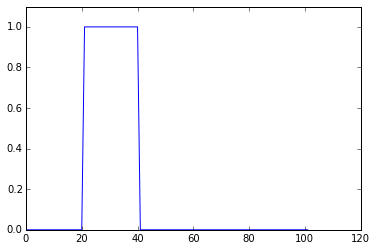In :
convection_solve(U, dx, dt, nx, len(t))
pcolormesh(U, rasterized=True, vmin=-2, vmax=2)

Out:
<matplotlib.collections.QuadMesh at 0x7f9ae56df978>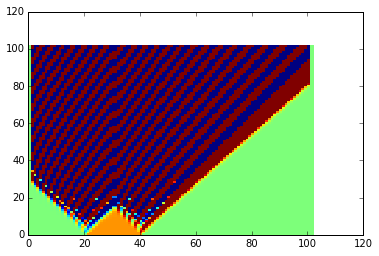:-( Does it help if we choose smaller time step?

In :
U, dx, dt, x, t = wave_init(maxx, maxt, v, nx, CFL=.1)
U[0,:] = 0.
U[0, (x<maxx*0.4) & (x>maxx*0.2)] = 1.
convection_solve(U, dx, dt, nx, len(t))
pcolormesh(U, rasterized=True, vmin=-2, vmax=2)

Out:
<matplotlib.collections.QuadMesh at 0x7f9ae52d1b00>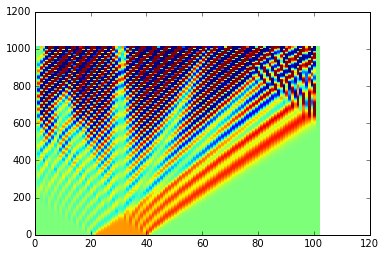Not really, this solver fails to reproduce the expected solution. See Von Neumann stability analysis or matrix method...

The Lax(-Friedrichs) method stabilizes the solution by replacing $U^n_i$ with centered approximation $\frac{1}{2}(U^n_{i-1}+U^n_{i+1})$: $$U^{n+1}_i = \frac{1}{2}(U^n_{i-1}+U^n_{i+1}) - \frac{c\Delta t}{2\Delta x}(U^n_{i+1}-U^n_{i-1})$$

In :
def convection_solve_Lax(U, dx, dt, nx, nt):
xint = arange(1, nx+1)
for it in range(0,nt-1):
U[it+1,xint] = 0.5*(U[it,xint+1]+U[it,xint-1]) - 0.5*v*dt/dx*(U[it,xint+1] - U[it, xint-1])

In :
U, dx, dt, x, t = wave_init(maxx, maxt, v, nx, CFL=1)
U[0,:] = 0.
U[0, (x<maxx*0.4) & (x>maxx*0.2)] = 1.
convection_solve_Lax(U, dx, dt, nx, len(t))
pcolormesh(U, rasterized=True, vmin=-2, vmax=2)

Out:
<matplotlib.collections.QuadMesh at 0x7f9ae5219668>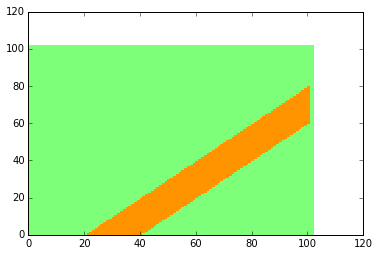The Courant-Friedrichs-Lewy (CFL) condition $$\frac{v\Delta t}{\Delta x}\leq 1$$ determines the stability. Look at the sensitivity to violating this condition:

In :
U, dx, dt, x, t = wave_init(maxx, maxt, v, nx, CFL=1.05)
U[0,:] = 0.
U[0, (x<maxx*0.4) & (x>maxx*0.2)] = 1.
convection_solve_Lax(U, dx, dt, nx, len(t))
pcolormesh(U, rasterized=True, vmin=-2, vmax=2)

Out:
<matplotlib.collections.QuadMesh at 0x7f9ae53e4e48>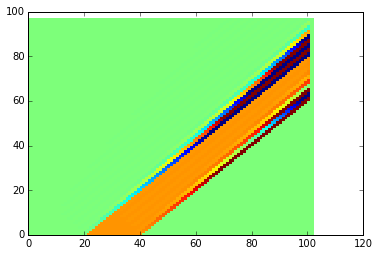What if we need better time resolution?

In :
U, dx, dt, x, t = wave_init(maxx, maxt, v, nx, CFL=.1)
U[0,:] = 0.
U[0, (x<maxx*0.4) & (x>maxx*0.2)] = 1.
convection_solve_Lax(U, dx, dt, nx, len(t))
pcolormesh(U, rasterized=True, vmin=-2, vmax=2)

Out:
<matplotlib.collections.QuadMesh at 0x7f9ae5158860>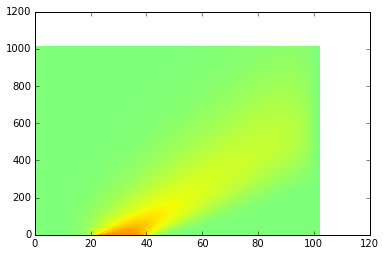The stabilization also causes "damping" of the solution at smaller time steps.

In :
def convection_solve_upwind(U, dx, dt, nx, nt):
xint = arange(1, nx+1)
for it in range(0,nt-1):
U[it+1,xint] = U[it,xint] - v*dt/dx*(U[it,xint] - U[it, xint-1])

In :
U, dx, dt, x, t = wave_init(maxx, maxt, v, nx, CFL=.1)
U[0,:] = 0.
U[0, (x<maxx*0.4) & (x>maxx*0.2)] = 1.
convection_solve_upwind(U, dx, dt, nx, len(t))
pcolormesh(U, rasterized=True, vmin=-2, vmax=2)

Out:
<matplotlib.collections.QuadMesh at 0x7f9ae1fc9ac8>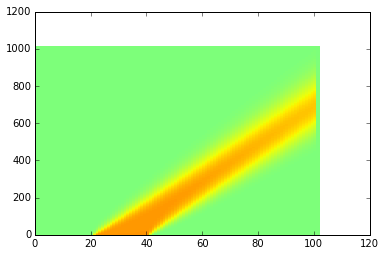In :
def convection_solve_LeapFrog(U, dx, dt, nx, nt):
xint = arange(1, nx+1)
#start the solution with upwind method
U[1,xint] = U[0,xint] - v*dt/dx*(U[0,xint] - U[0, xint-1])
for it in range(1, nt-1):
U[it+1,xint] = U[it-1,xint] - v*dt/dx*(U[it,xint+1] - U[it, xint-1])

U, dx, dt, x, t = wave_init(maxx, maxt, v, nx, CFL=.1)
U[0,:] = 0.
U[0, (x<maxx*0.4) & (x>maxx*0.2)] = 1.
convection_solve_LeapFrog(U, dx, dt, nx, len(t))
pcolormesh(U, rasterized=True, vmin=-2, vmax=2)

Out:
<matplotlib.collections.QuadMesh at 0x7f9ae509bcc0>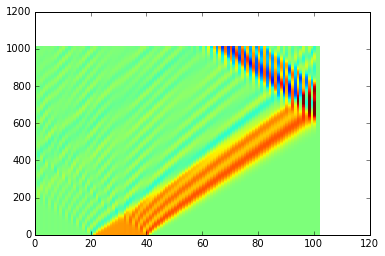In :
def convection_solve_LeapFrog(U, dx, dt, nx, nt):
xint = arange(1, nx+1)
#start the solution with upwind method
U[1,xint] = U[0,xint] - v*dt/dx*(U[0,xint] - U[0, xint-1])
for it in range(1, nt-1):
U[it+1,xint] = U[it-1,xint] - v*dt/dx*(U[it,xint+1] - U[it, xint-1])

#extrapolate values on the outflow boundary
U[it+1,-1] = U[it+1, -2]
U, dx, dt, x, t = wave_init(maxx, maxt, v, nx, CFL=1)
U[0,:] = 0.
U[0, (x<maxx*0.4) & (x>maxx*0.2)] = 1.
convection_solve_LeapFrog(U, dx, dt, nx, len(t))
pcolormesh(U, rasterized=True, vmin=-2, vmax=2)

Out:
<matplotlib.collections.QuadMesh at 0x7f9ae201d358>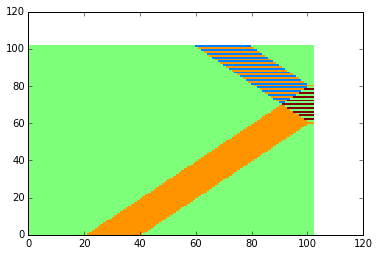In :
def convection_solve_LeapFrog(U, dx, dt, nx, nt):
xint = arange(1, nx+1)
#start and smooth the solution with upwind method
for it in range(0,min(50, nt-1)):
U[it+1,xint] = U[it,xint] - v*dt/dx*(U[it,xint] - U[it, xint-1])
#U[it+1,xint] = 0.5*(U[it,xint+1]+U[it,xint-1]) - 0.5*v*dt/dx*(U[it,xint+1] - U[it, xint-1])
for it in range(min(50, nt-1),nt-1):
U[it+1,xint] = U[it-1,xint] - v*dt/dx*(U[it,xint+1] - U[it, xint-1])

#extrapolate values on the outflow boundary
U[it+1,-1] = U[it+1, -2]*2 - U[it+1, -3]
U, dx, dt, x, t = wave_init(maxx, maxt, v, nx, CFL=1)
U[0,:] = 0.
U[0, (x<maxx*0.4) & (x>maxx*0.2)] = 1.
convection_solve_LeapFrog(U, dx, dt, nx, len(t))
pcolormesh(U, rasterized=True, vmin=-2, vmax=2)

Out:
<matplotlib.collections.QuadMesh at 0x7f9ae1fcf908>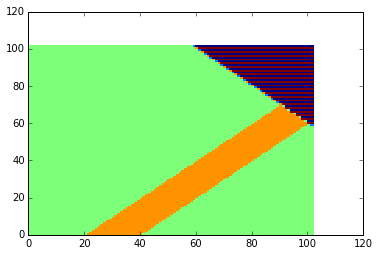In [ ]:
def convection_solve_LaxWendroff(U, dx, dt, nx, nt):
xint = arange(1, nx+1)
for it in range(0,nt-1):
# less efficient but more readable:
Uplus  = 0.5*(U[it,xint+1] + U[it, xint]) - 0.5*v*dt/dx*(U[it,xint+1] - U[it, xint])
Uminus = 0.5*(U[it,xint] + U[it, xint-1]) - 0.5*v*dt/dx*(U[it,xint] - U[it, xint-1])
U[it+1,xint] = U[it,xint] - v*dt/dx*(Uplus - Uminus)

In [ ]:
U, dx, dt, x, t = wave_init(maxx, maxt, v, nx, CFL=.1)
U[0,:] = 0.
U[0, (x<maxx*0.4) & (x>maxx*0.2)] = 1.
convection_solve_LaxWendroff(U, dx, dt, nx, len(t))
pcolormesh(U, rasterized=True, vmin=-2, vmax=2)

In [ ]:
def convection_solve_LaxWendroff(U, dx, dt, nx, nt):
xint = arange(1, nx+1)
for it in range(0,nt-1):
# less efficient but more readable:
Uplus  = 0.5*(U[it,xint+1] + U[it, xint]) - 0.5*v*dt/dx*(U[it,xint+1] - U[it, xint])
Uminus = 0.5*(U[it,xint] + U[it, xint-1]) - 0.5*v*dt/dx*(U[it,xint] - U[it, xint-1])
U[it+1,xint] = U[it,xint] - v*dt/dx*(Uplus - Uminus)

#extrapolate values on the outflow boundary
U[it+1,-1] = U[it+1, -2]*2 - U[it+1,-3]

In [ ]:
U, dx, dt, x, t = wave_init(maxx, maxt, v, nx, CFL=.1)
U[0,:] = 0.
U[0, (x<maxx*0.4) & (x>maxx*0.2)] = 1.
convection_solve_LaxWendroff(U, dx, dt, nx, len(t))
pcolormesh(U, rasterized=True, vmin=-2, vmax=2)

In [ ]:
def convection_solve_LaxWendroff(U, dx, dt, nx, nt):
xint = arange(1, nx+1)
for it in range(0,min(50, nt-1)):
U[it+1,xint] = U[it,xint] - v*dt/dx*(U[it,xint] - U[it, xint-1])
for it in range(min(50, nt-1),nt-1):
# less efficient but more readable:
Uplus  = 0.5*(U[it,xint+1] + U[it, xint]) - 0.5*v*dt/dx*(U[it,xint+1] - U[it, xint])
Uminus = 0.5*(U[it,xint] + U[it, xint-1]) - 0.5*v*dt/dx*(U[it,xint] - U[it, xint-1])
U[it+1,xint] = U[it,xint] - v*dt/dx*(Uplus - Uminus)

#extrapolate values on the outflow boundary
U[it+1,-1] = U[it+1, -2]*2 - U[it+1,-3]

U, dx, dt, x, t = wave_init(maxx, maxt, v, nx, CFL=.1)
U[0,:] = 0.
U[0, (x<maxx*0.4) & (x>maxx*0.2)] = 1.
convection_solve_LaxWendroff(U, dx, dt, nx, len(t))
pcolormesh(U, rasterized=True, vmin=-2, vmax=2)


Can we break the CFL condition with some naive implementation of implicit scheme?

In [ ]:
from scipy.sparse import dia_matrix, eye
from scipy.sparse.linalg import factorized
def d1matrix(nelem):
elements = ones((3,nelem))
elements[1,:] *= 0
elements[0,:] *= -1
return dia_matrix((elements, [-1,0,1]), shape=(nelem,nelem)).tocsc()

def convection_solve_CN(U, dx, dt, nx, nt):
alpha = -v*dt/(dx*4.)
M1 = eye(nx)-d1matrix(nx)*alpha
M2 = eye(nx)+d1matrix(nx)*alpha
LU = factorized(M1.tocsc())
for it in range(0,nt-1):
U[it+1,1:-1] = LU(M2.dot(U[it,1:-1]))

#extrapolate values on the outflow boundary
U[it+1,-1] = U[it+1, -2]


In [ ]:
U, dx, dt, x, t = wave_init(maxx, maxt, v, nx, CFL=2)
U[0,:] = 0.
U[0, (x<maxx*0.4) & (x>maxx*0.2)] = 1.
convection_solve_CN(U, dx, dt, nx, len(t))
pcolormesh(U, rasterized=True, vmin=-2, vmax=2)


It sort of works, but not very well...

In [ ]: